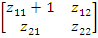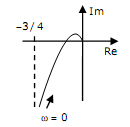# x(t) is a positive rectangular pulse from t=-1 to t=+1 with unit height as shown in the figure.The value of  {where X(ω) is the fourier transform of x(t)} is

1.  2

2.  2π

3.  4

4.  4π

4

Explanation :
No Explanation available for this question

# Given the finite length input x[n] and the corresponding finite length output y[n] of an LTI system as shown below, the impulse response h[n] of the system is

1.  h [n] = {1,0,0,1}

2.  h [n] = {1,0,1}

3.  h [n] = {1,1,1,1}

4.  h [n] = {1,1,1}

4

h [n] = {1,1,1,1}

Explanation :
No Explanation available for this question

# If the 12Ω resistor draws a current of 1A as shown in the figure, the value of resistance R is

1.  4Ω

2.  6Ω

3.  8Ω

4.  18Ω

4

Explanation :
No Explanation available for this question

# The two-port network P shown in the figure has ports 1 and 2,denoted by terminals (a,b) and (c,d), respectively. It has an impedance matrix Z with parameters denoted by zij.A 1Ω resistor is connected in series with the network at port 1 as shown in the figure.The impedance matrix of the modified two-port network (shown as a dashed box) is

1.

2.

3.

4.

4Explanation :
No Explanation available for this question

# The Maxwell's bridge shown in the figure is at balance.The parameters of the inductive coil are

1.  R = R2R3/R4, L = C4R2R3

2.  L= R2R3/R4, R= C4R2R3

3.  R = R4/R2R3, L = 1 (C4R2R3)

4.  L = R4/R2R3, R = 1/ (C4R2R3)

4

R = R2R3/R4, L = C4R2R3

Explanation :
No Explanation available for this question

# The frequency response of G(s) = 1/ [s(s +1)(s + 2)] plotted in the complex G(jω) plane (for 0

1.

2.

3.

4.

4Explanation :
No Explanation available for this question

# The system x = Ax + Bu with

1.  stable and controllable

2.  stable but uncontrollable

3.  unstable but controllable

4.  unstable and uncontrollable

4

unstable but controllable

Explanation :
No Explanation available for this question

# The characteristic equation of a closed-loop system s(s+1)(s+3)+k(s+2)=0,k>0.Which of the following statements is true

1.  Its roots are always real

2.   It cannot have a breakaway point in the range -1 < Re[s] < 0

3.  Two of its roots tend to infinity along the asymptotes Re[s] = -1

4.  It may have complex roots in the right half plane is

4

Two of its roots tend to infinity along the asymptotes Re[s] = -1

Explanation :
No Explanation available for this question

# A separately excited dc machine is coupled to a 50Hz, three-phase, 4-pole induction machine as shown in the figure. The dc machine is energized first and the machines rotate at 1600 rpm. Subsequently the induction machine is also connected to a 50Hz, three-phase source, the phase sequence being consistent with the direction of rotation. In steady state,

1.   Both machines act as generators

2.  The dc machine acts as a generator, and the induction machine acts as a motor

3.  The dc machine acts as a motor, and the induction machine acts as a generator

4.  Both machines act as motors

4

The dc machine acts as a motor, and the induction machine acts as a generator

Explanation :
No Explanation available for this question

# A balanced star-connected and purely resistive load is connected at the secondary of a star-delta transformer as shown in the figure.The line-to-line voltage rating of the transformer is 110V/220V.Neglecting the non-idealities of the transformer,the impedance 'Z' of the equivalent star-connected load,referred to the primary side of the transformer,is

1.  (3+j0)Ω

2.   (0.866-j0.5)Ω

3.   (0.866+j0.5)Ω

4.  (1+j0)Ω

4